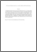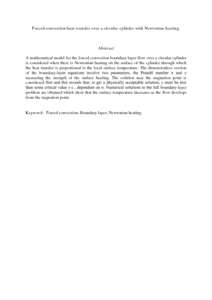# Forced-convection heat transfer over a circular cylinder with Newtonian heating.

## Citation

Salleh, M. Z. and Nazar, Roslinda and Md. Arifin, Norihan and Pop, Ioan and Merkin, J. H. (2011) Forced-convection heat transfer over a circular cylinder with Newtonian heating. Journal of Engineering Mathematics , 69 (1). pp. 101-110. ISSN 0022-0833; ESSN:1573-2703

## Abstract

A mathematical model for the forced convection boundary-layer flow over a circular cylinder is considered when there is Newtonian heating on the surface of the cylinder through which the heat transfer is proportional to the local surface temperature. The dimensionless version of the boundary-layer equations involve two parameters, the Prandtl number σ and γ measuring the strength of the surface heating. The solution near the stagnation point is considered first and this reveals that, to get a physically acceptable solution, γ must be less than some critical value γ c , dependent on σ. Numerical solutions to the full boundary-layer problem are obtained which show that the surface temperature increases as the flow develops from the stagnation point.Preview
PDF (Abstract)
Forced-convection heat transfer over a circular cylinder with Newtonian heating..pdfView Item